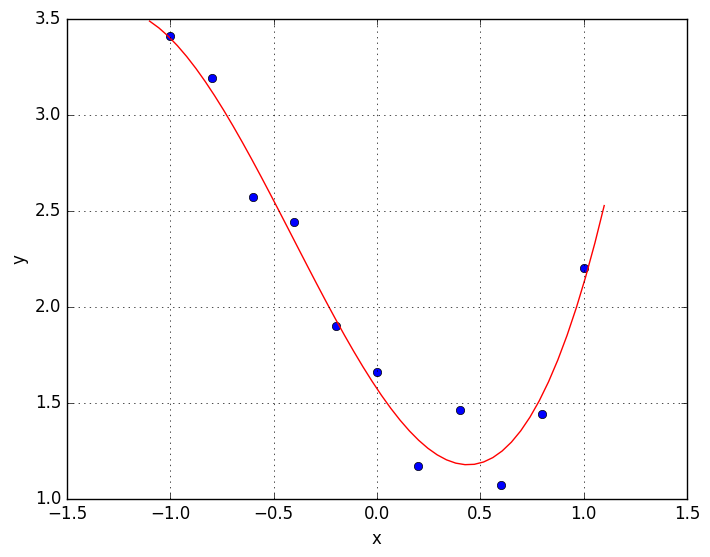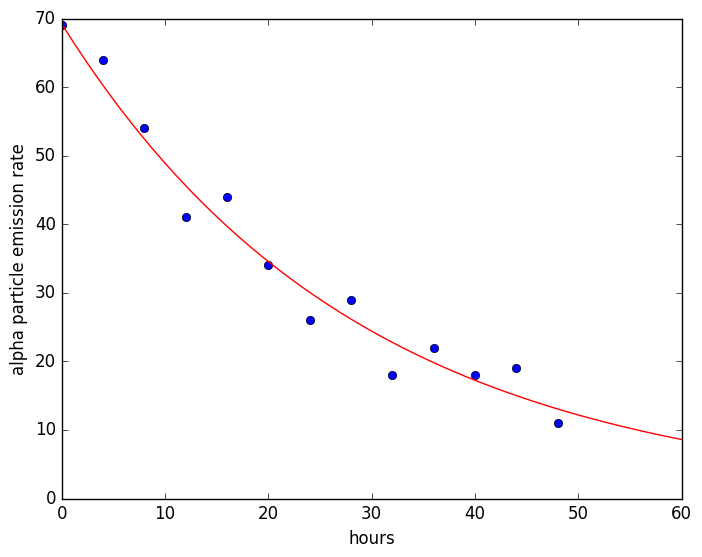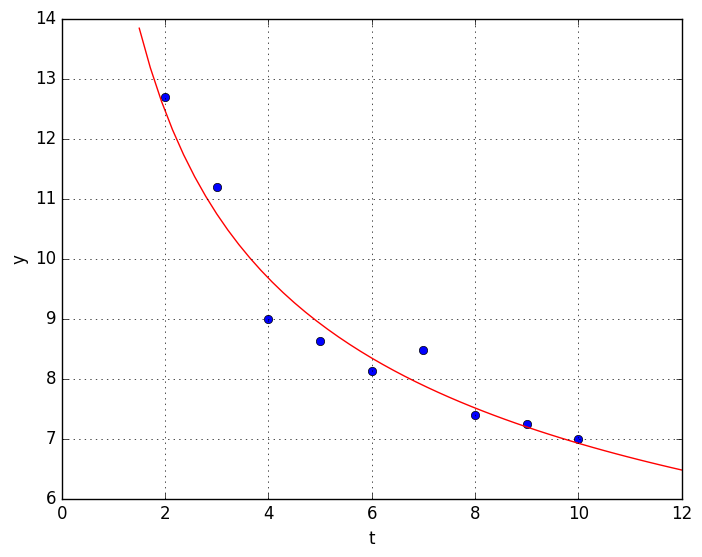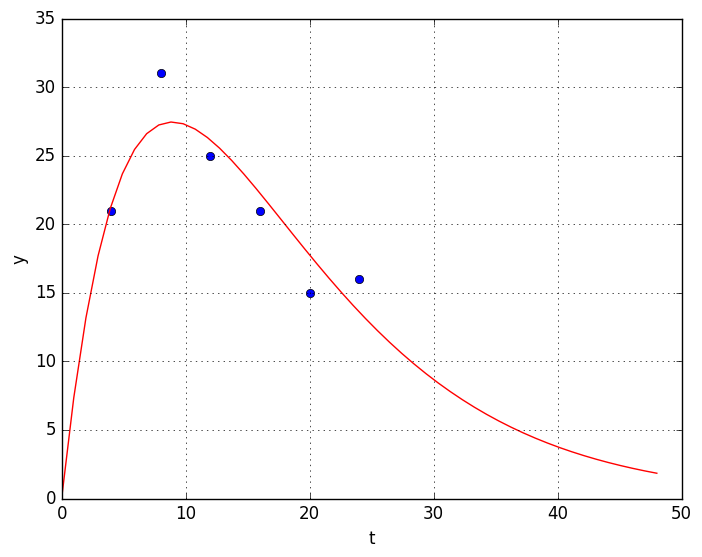# Solutions¶

### Problem 1. Polynomial least-squares fit¶

(a) Fit a cubic polynomial to the following data, and make a plot that shows the data as dots and the cubic fit as a smooth curve.

In :
xdata = [-1.0,  -0.8,  -0.6,  -0.4,  -0.2,  0.0,  0.2,  0.4,  0.6,  0.8,  1.0];
ydata = [3.41, 3.19,  2.57,  2.44,  1.90, 1.66, 1.17, 1.46, 1.07, 1.44, 2.2];

In :
# Set up Vandermonde matrix
m = length(xdata)
n = 4
X = zeros(m,n)
X[:,1] = 1
for j = 2:n
X[:,j] = X[:,j-1] .* xdata
end

# Solve least-sqaures system X c = ydata. I'm going to use Julia's
# backslash operater because I am confident of my understanding of
# what's going on behind the scenes (least squares via QR decomp)
c = X\ydata

Out:
4-element Array{Float64,1}:
1.57247
-1.61084
1.18473
0.97028
In :
function polyeval(c,x)
N = length(c);
p = c[N]
for n = N-1:-1:1
p = p.*x + c[n]
end
p
end

using PyPlot
plot(xdata, ydata, "bo", label="datapoints")

x=linspace(-1.1, 1.1)
plot(x, polyeval(c,x), "r-", label="least-squares cubic fit")
xlabel("x")
ylabel("y")
grid("on")(b) Write out the polynomial in the form $P(x) = c_0 + c_1 x + c_2 x^2 + c_3 x^3$ with the coefficients specified as numeric values with three digits.

In :
c

Out:
4-element Array{Float64,1}:
1.57247
-1.61084
1.18473
0.97028
In :
P(x) = 1.57 - 1.61 x + 1.18 x^2 + 0.97 x^3

LoadError: syntax: extra token "x" after end of expression


### Problem 2. Exponential fit¶

(a) Given the following experimental measurements of alpha-particle emission of a radioactive substance, fit an exponential function $y = c \exp(a t)$ to the data using least squares. Make a plot that shows the data as dots and the exponential fit as a smooth curve. What are the values of $c$ and $a$?

In :
tdata = [0,   4,  8, 12, 16, 20, 24, 28, 32, 36, 40, 44, 48];
ydata = [69, 64, 54, 41, 44, 34, 26, 29, 18, 22, 18, 19, 11];


First we take the log of $y = c e^{at}$ to get

\begin{equation*} \log y = \log c + a t \end{equation*}

The linear system for a bunch of $(t_i,y_i)$ data points is then

\begin{equation*} \left[\begin{array}{cc} 1 & t_1 \\ 1 & t_2 \\ 1 & t_3 \\ \vdots & \vdots \end{array} \right] \left[\begin{array}{c} \log c \\ a \end{array}\right] = \left[\begin{array}{c} \log y_1 \\ \log y_2 \\ \log y_3 \\ \vdots \end{array} \right] \end{equation*}

Call that $T x = \log y$, where $x_1 = \log c$ and $x_2 = a$. Do the calculation in Julia.

In :
m = length(tdata)
T = zeros(m,2)
T[:,1] = 1
T[:,2] = tdata
x = T\log(ydata)
c = exp(x)
a = x

plot(tdata, ydata, "bo", label="datapoints")
t = linspace(0, 60)
plot(t, c*exp(a*t), "r-", label="least-squares exponential")
xlabel("hours")
ylabel("alpha particle emission rate")Out:
PyObject <matplotlib.text.Text object at 0x7fef67d23f10>
In :
c, a

WARNING: Base.writemime is deprecated.
likely near /home/gibson/.julia/v0.5/IJulia/src/kernel.jl:31
WARNING: Base.writemime is deprecated.
likely near /home/gibson/.julia/v0.5/IJulia/src/kernel.jl:31
WARNING: Base.writemime is deprecated.
likely near /home/gibson/.julia/v0.5/IJulia/src/kernel.jl:31
WARNING: Base.writemime is deprecated.
likely near /home/gibson/.julia/v0.5/IJulia/src/kernel.jl:31
WARNING: Base.writemime is deprecated.
likely near /home/gibson/.julia/v0.5/IJulia/src/kernel.jl:31
WARNING: Base.writemime is deprecated.
likely near /home/gibson/.julia/v0.5/IJulia/src/kernel.jl:31
WARNING: Base.writemime is deprecated.
likely near /home/gibson/.julia/v0.5/IJulia/src/kernel.jl:31
WARNING: Base.writemime is deprecated.
likely near /home/gibson/.julia/v0.5/IJulia/src/kernel.jl:31
WARNING: Base.writemime is deprecated.
likely near /home/gibson/.julia/v0.5/IJulia/src/kernel.jl:31
WARNING: Base.writemime is deprecated.
likely near /home/gibson/.julia/v0.5/IJulia/src/kernel.jl:31
WARNING: Base.writemime is deprecated.
likely near /home/gibson/.julia/v0.5/IJulia/src/kernel.jl:31
WARNING: Base.writemime is deprecated.
likely near /home/gibson/.julia/v0.5/IJulia/src/kernel.jl:31
WARNING: Base.writemime is deprecated.
likely near /home/gibson/.julia/v0.5/IJulia/src/kernel.jl:31
in show at /home/gibson/.julia/v0.5/PyCall/src/PyCall.jl
WARNING: Base.writemime is deprecated.
likely near /home/gibson/.julia/v0.5/IJulia/src/kernel.jl:31
in show at /home/gibson/.julia/v0.5/PyCall/src/PyCall.jl
WARNING: Base.writemime is deprecated.
likely near /home/gibson/.julia/v0.5/IJulia/src/kernel.jl:31
in show at /home/gibson/.julia/v0.5/PyCall/src/PyCall.jl
WARNING: Base.writemime is deprecated.
likely near /home/gibson/.julia/v0.5/IJulia/src/kernel.jl:31
in show at /home/gibson/.julia/v0.5/PyCall/src/PyCall.jl
WARNING: Base.writemime is deprecated.
likely near /home/gibson/.julia/v0.5/IJulia/src/kernel.jl:31
in show at /home/gibson/.julia/v0.5/PyCall/src/PyCall.jl
WARNING: Base.writemime is deprecated.
likely near /home/gibson/.julia/v0.5/IJulia/src/kernel.jl:31
in show at /home/gibson/.julia/v0.5/PyCall/src/PyCall.jl
WARNING: Base.writemime is deprecated.
likely near /home/gibson/.julia/v0.5/IJulia/src/kernel.jl:31
in show at /home/gibson/.julia/v0.5/PyCall/src/PyCall.jl
WARNING: Base.writemime is deprecated.
likely near /home/gibson/.julia/v0.5/IJulia/src/kernel.jl:31
in show at /home/gibson/.julia/v0.5/PyCall/src/PyCall.jl
WARNING: Base.writemime is deprecated.
likely near /home/gibson/.julia/v0.5/IJulia/src/kernel.jl:31
in show at /home/gibson/.julia/v0.5/PyCall/src/PyCall.jl
WARNING: Base.writemime is deprecated.
likely near /home/gibson/.julia/v0.5/IJulia/src/kernel.jl:31
in show at /home/gibson/.julia/v0.5/PyCall/src/PyCall.jl
WARNING: Base.writemime is deprecated.
likely near /home/gibson/.julia/v0.5/IJulia/src/kernel.jl:31
in show at /home/gibson/.julia/v0.5/PyCall/src/PyCall.jl
WARNING: Base.writemime is deprecated.
likely near /home/gibson/.julia/v0.5/IJulia/src/kernel.jl:31
in show at /home/gibson/.julia/v0.5/PyCall/src/PyCall.jl

Out:
(69.23564314813459,-0.03475031092464803)
In :
# So the exponential fit is
f(t) = 69.2 * exp(-0.348*t)

Out:
f (generic function with 1 method)

(b) What is the substance's half-life? (i.e. the time $t$ for which $y(t)/y(0) = 1/2$)

In :
# for  y(t) = c exp(at), y(0) = c, so we have
#      y(t) = y(0) exp(at)
# y(t)/y(0) = exp(at)

# Thus if y(t)/y(0) = 1/2 = exp(at),
# log 1/2 = at, and
# the halflife is given by t = 1/a log 1/2
t = 1/a * log(1/2)

Out:
19.946502984187784
In :
# Looks like the half-life of the substance is about 20 hrs.


### Problem 3. Power-law fit¶

(a) Fit a power-law curve $y = c t^a$ to the following data, and make a plot showing the datapoints as dots and the fit as a smooth curve.

In :
tdata =  [   2,    3,    4,    5,    6,    7,    8,    9,   10];
ydata =  [12.7, 11.2, 8.99, 8.62, 8.12, 8.47, 7.39, 7.24, 6.99];


Take the log of $y = c t^a$ to get

\begin{equation*} \log y = \log c + a \log t \end{equation*}

The linear system for a bunch of $(t_i,y_i)$ data points is then

\begin{equation*} \left[\begin{array}{cc} 1 & \log t_1 \\ 1 & \log t_2 \\ 1 & \log t_3 \\ \vdots & \vdots \end{array} \right] \left[\begin{array}{c} \log c \\ a \end{array}\right] = \left[\begin{array}{c} \log y_1 \\ \log y_2 \\ \log y_3 \\ \vdots \end{array} \right] \end{equation*}

Call that $T x = \log y$, where $x_1 = \log c$ and $x_2 = a$.

Now do the calculation in Julia.

In :
m = length(tdata)
T = zeros(m,2)
T[:,1] = 1
T[:,2] = log(tdata)
x = T\log(ydata)
c = exp(x)
a = x

plot(tdata, ydata, "bo", label="datapoints")
t = linspace(1.5, 12)
plot(t, c*t.^a, "r-", label="least-squares power law")
xlabel("t")
ylabel("y")
grid("on")(b) Write out the least-squares power-law fit $y = c t^a$ with $c$ and $a$ specified as numeric values with three digits.

In :
c, a

Out:
(16.04696670297259,-0.3652492064261485)
In :
y(t) = 16.05 * t^-0.365

Out:
y (generic function with 1 method)

### Problem 4. c t exp(at) fit¶

(a) The following data represent measurements of blood concentration of a drug after intravenous injection as a function of time. Fit a function of the form $y = c \, t \, e^{at}$ to the data using least squares. Make a plot that shows the data as dots and the exponential fit as a smooth curve.

In :
# t is time in hours, y is concentration in ng/ml
tdata = [4,   8,  12,  16,  20,  24];
ydata = [21, 31,  25,  21,  15,  16];


Rewrite $y = c t e^{at}$ as $y/t = c e^{at}$ and then take the log of both sides to get

\begin{equation*} \log y/t = \log c + a t \end{equation*}

The linear system for a bunch of $(t_i,y_i)$ data points is then

\begin{equation*} \left[\begin{array}{cc} 1 & t_1 \\ 1 & t_2 \\ 1 & t_3 \\ \vdots & \vdots \end{array} \right] \left[\begin{array}{c} \log c \\ a \end{array}\right] = \left[\begin{array}{c} \log y_1/t_1 \\ \log y_2/t_2 \\ \log y_3/t_3 \\ \vdots \end{array} \right] \end{equation*}

Call that $A x = \log y/t$, where $x_1 = \log c$ and $x_2 = a$.

Now do the calculation in Julia.

In :
m = length(tdata)
A = zeros(m,2)
A[:,1] = 1
A[:,2] = tdata
x = A\log(ydata./tdata)
c = exp(x)
a = x

plot(tdata, ydata, "bo", label="datapoints")
t = linspace(0, 48)
plot(t, c*t.*exp(a*t), "r-", label="least-squares ct exp(at) fit")
xlabel("t")
ylabel("y")
grid("on")(b) Write out the model $y = c \, t \, e^{at}$ with numeric values specified to three digits.

In :
c, a

Out:
(8.372674496790392,-0.11219417563518795)
In :
y(t) = 8.37*t * exp(-0.112*t)

WARNING: Method definition y(Any) in module Main at In:1 overwritten at In:1.

Out:
y (generic function with 1 method)

(c) Based on the model, at what time do you expect the concentration to reach 5 ng/ml?

In :
# Looks like about 37 hrs from the graph. Could try to solve the nonlinear equation for t,
# i.e. 5 = 8.37 t exp(-0.112 t), but that would be overkill, given the approximate nature
# of the least-squares fit.